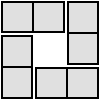#### You may also like### 4 Dom

Use these four dominoes to make a square that has the same number of dots on each side.### Just Rolling Round

P is a point on the circumference of a circle radius r which rolls, without slipping, inside a circle of radius 2r. What is the locus of P?### Areas and Ratios

Do you have enough information to work out the area of the shaded quadrilateral?

# Mapping the Territory

##### Age 14 to 18Challenge Level

This resource is part of our Adventures with Complex Numbers collection

This activity follows on from Complex Puzzle.

Use the Geogebra interactivity below to find some pairs of complex numbers whose product is a real number. What do you notice?
Can you explain it algebraically?

Use the Geogebra interactivity to find some pairs of complex numbers whose product is an imaginary number. What do you notice?
Can you explain it algebraically?

In general, what would you need to multiply by $a+bi$ to get a real number?  Or to get an imaginary number?

For a given complex number $a + bi$, what would you need to multiply by to get to another given number $x + yi$?
How does this relate to your geometric interpretation of multiplication of complex numbers?